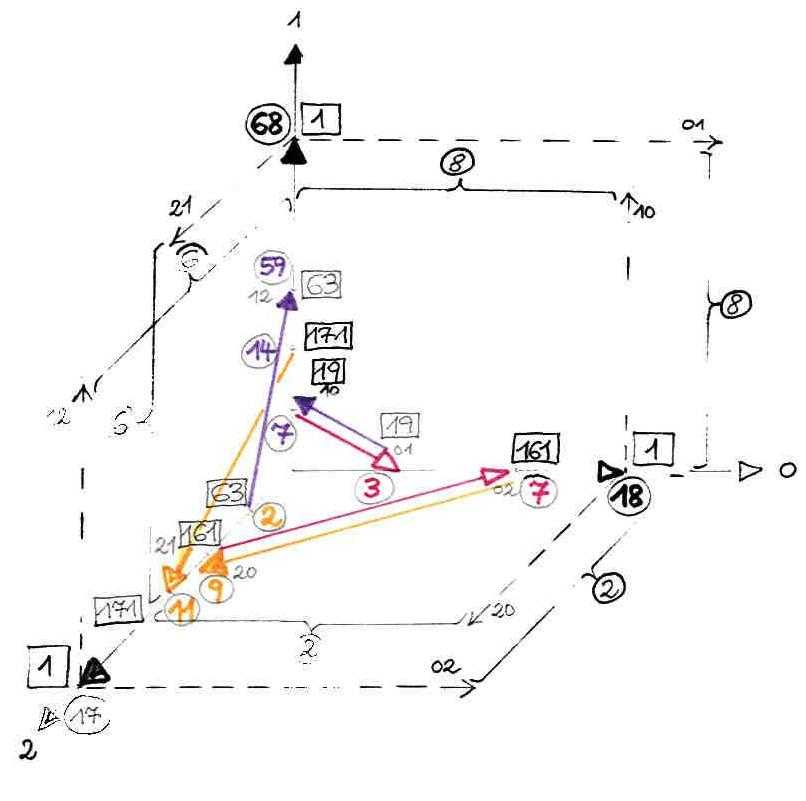# of Karim Belabas, Aïssa Derhem, and Daniel C. Mayer

 * On these pages, we present most recent results of our joint research, directly from the lab. * Basic bibliography: K. Belabas, A fast algorithm to compute cubic fields, Math. Comp. 66 (1997), 1213-1237 A. Derhem, Capitulation dans les extensions quadratiques de corps de nombres cubiques cycliques, Thèse de doctorat, Université Laval, Quebec, 1988 D. C. Mayer, Multiplicities of dihedral discriminants, Math. Comp. 58 (1992), 831-847 and S55-S58 * E-mail addresses: Karim.Belabas@math.u-psud.fr aderhem@yahoo.fr danielmayer@algebra.at *

 Geometry of Totally Real Cubic Fields (2002/03/23) Dan (02/03/15): Now I have tuned the VORONOI algorithm for Signature (3,0) to run with 4th generation languages (4GL: Java, C++, Delphi). As a first trial, I have analyzed the totally real cubic field with discriminant d = 5768321, Dan (02/03/16): Johannes Buchmann has chosen this field to demonstrate the power of the algorithms presented in his Habilitations-Schrift 1987 at the University of Düsseldorf.The discriminant lies far outside the range d < 5*10^5 of Ennola and Turunen's tables from 1985, which contain the most recent computer calculated regulators and fundamental systems of units. (Llorente and Quer have computed a larger table in 1988. It covers the range d < 10^7, but it doesn't give regulators and units.) Dan (02/03/23): 1. Buchmann starts with the generating polynomial P(X) = X^3 - 113 X - 11 P(X) has 3 real zeros: xi = xi_1 = 10.678488112627206... xi_2 = -10.581134814557196... xi_3 = -0.09735329807001065... I determined an integral basis with the aid of Voronoi's master's thesis: (om_1, om_2, om_3) = ( 1, xi, xi^2 - 113 ) Hence, the maximal order of the totally real cubic L = Q(xi) is O_L = Z*om_1 + Z*om_2 + Z*om_3 By LLL-reduction of the power basis, Buchman gets another integral basis (om_1, om_2, om_3) = ( 1, xi, xi^2 - 75 ) The polynomial index is i(P) = 1, whence polynomial discriminant and field discriminant coincide: d(P) = d_L = 5768321 = 13*17*43*607 The discriminant d_L being squarefree, we get the conductor f = 1, i. e., the normal field N of L is an unramified Hilbert class field over its quadratic subfield K with discriminant d = d_L. Indeed, counting cycles of reduced indefinite quadratic forms yields the class number h = 48 = 2^4*3 and thus the 3-class rank rho = 1 of K. The fundamental unit of K is rather big eta = 19245990076771983019414359282821719387667305+ +8013376486711217763609247832156537986212*d^1/2 the regulator is r = 100.3590238176445... and the period length l = 84, as the continued fraction algorithm shows. Of course, the Principal Factorization Type of L is DELTA1, i. e., we have a 1-dimensional capitulation of the class of K of order 3 in N and eta arises as the norm of a unit in N; 2. Using VORONOI's algorithm for signature (3,0) of his doctoral dissertation, I determined the geometry of lattice minima in the discrete geometric image of O_L, the maximal order of Buchmann's totally real cubic: Chain in X-direction: no pre period, chain length l_X = 18, N(n_X^18(1)) = 1 X-Branch in Y-direction: branch length l_XY = 7, association on X-chain at pos = 3, N(n_Y^7(1)) = N(n_X^3(1)) = 19 X-Branch in Z-direction: branch length l_XZ = 9, association on X-chain at pos = 7, N(n_Z^9(1)) = N(n_X^7(1)) = 161 Chain in Y-direction: no pre period, chain length l_Y = 68, N(n_Y^68(1)) = 1 Y-Branch in Z-direction: branch length l_YZ = 2, association on Y-chain at pos = 59, N(n_Z^2(1)) = N(n_Y^59(1)) = 63 Y-Branch in X-direction: branch length l_YX = 3, association on Y-chain at pos = 7, N(n_X^3(1)) = N(n_Y^7(1)) = 19 Chain in Z-direction: no pre period, chain length l_Z = 17, N(n_Z^17(1)) = 1 Z-Branch in X-direction: branch length l_ZX = 7, association on Z-chain at pos = 9, N(n_X^7(1)) = N(n_Z^9(1)) = 161 Z-Branch in Y-direction: branch length l_ZY = 14, association on Z-chain at pos = 11, N(n_Y^14(1)) = N(n_Z^11(1)) = 171Regulator R = 506.889707164989... None of the DIRICHLET systems of units is fundamental: XY-system: index ( U : < e_X,e_Y > ) = 8 YZ-system: index ( U : < e_Y,e_Z > ) = 6 ZX-system: index ( U : < e_Z,e_X > ) = 2 Of course, I got 6 fundamental pairs of units by VORONOI's algorithm: (e_X,e_XY),(e_X,e_XZ),(e_Y,e_YZ),(e_Y,e_YX),(e_Z,e_ZX),(e_Z,e_ZY) and I list the one with most similarity to Buchmann's result: e_X = 31 + 37xi + 43xi^2 1/e_XY = e_YX = -286276 - 2913780xi + 275375xi^2 = u_1 of Buchmann Buchmann gives the following fundamental pair of units w. r. to his LLL-basis: u_1 = 20366849om_1 - 2913780om_2 + 275375om_3 u_2 = 11501336om_1 - 346225om_2 + 153820om_3 3. Buchmann was able to determine the generalized 2-dimensional period length of Min(O_L), i. e., the number of orbits of U in Min(O_L): #(Min(O_L) / U) = 435 and he also gives the class number of L: h_L = 1